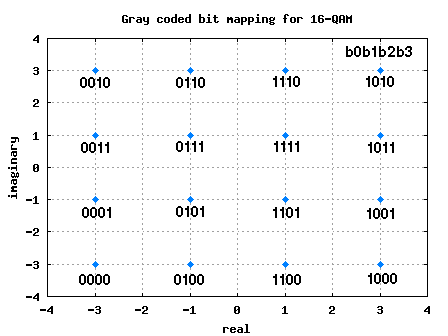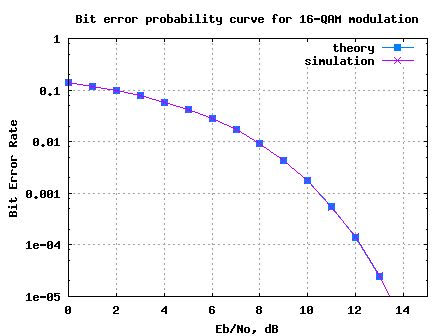- DSP log - http://www.dsplog.com -

16QAM Bit Error Rate (BER) with Gray mapping

Posted By Krishna Sankar On June 5, 2008 @ 6:06 am In Modulation | 79 Comments

Let us derive the theoretical 16QAM bit error rate (BER) with Gray coded constellation mapping in additive white Gaussian noise conditions. Further, the Matlab/Octave simulation script can be used to confirm that the simulation is in good agreement with theory.

Gray coded bit mapping in 16-QAM modulation

As we discussed in the previous post on Binary to Gray code for 16QAM , the 4 bits in each constellation point can be considered as two bits each on independent 4-PAM modulation on I-axis and Q-axis respectively.

 b0b1 I b2b3 Q 00 -3 00 -3 01 -1 01 -1 11 +1 11 +1 10 +3 10 +3

Table: Gray coded constellation mapping for 16-QAMFigure: 16QAM constellation plot with Gray coded mapping

Symbol Error and Bit Error probability

As can be seen from the above constellation diagram, with Gray coded bit mapping, adjacent constellation symbols differ by only one bit. So, if the noise causes the constellation to cross the decision threshold, only 1 out of bits will be in error. So the relation between bit error and symbol error is,

.

Note:

For very low value of , it may so happen that the noise causes the constellation to fall near a diagonally located constellation point. In that case, the each symbol error will cause two bit errors. Hence the need for approximate operator in the above equation. However, for reasonably high value of , the chances of such events are negligible.

Bit energy and symbol energy

As we learned from the post discussing Bit error rate for 16PSK , since each symbol consists of bits, the symbol to noise ratio k times the bit to noise ratio i.e,

where,

.

16QAM BER

From the post detaling the derivation of 16QAM Symbol error rate , we know that the symbol error is,

.

Combining the above two equations, the bit error rate for Gray coded 16QAM in Additive White Gaussian Noise is

.

Simulation model

The Matlab/Octave script performs the following:

(a) Generation of random binary sequence

(b) Assigning group of 4 bits to each 16-QAM constellation symbol per the Gray mapping

(c) Addition of white Gaussian Noise

(d) Demodulation of 16-QAM symbols and

(e) De-mapping per decimal to Gray conversion

(f) Counting the number of bit errors

(g) Running this for each value of Eb/No in steps of 1dB.Figure: Bit Error Rate plot for 16QAM modulation with Gray mapping

I think we have analyzed the bit error and symbol error probabilites for most of the PSK and QAM modulation schemes in Additive White Gaussian Noise. Time to move on to error rate in multipath channel. receiver diversity. MIMO channel, modulation with memory etc etcHope this helps,
Krishna

URL to article: http://www.dsplog.com/2008/06/05/16qam-bit-error-gray-mapping/

URLs in this post:

 Binary to Gray code for 16QAM: http://www.dsplog.com/2008/06/01/binary-to-gray-code-for-16qam/

 Bit error rate for 16PSK: http://www.dsplog.com/2008/05/18/bit-error-rate-for-16psk-modulation-using-gray-mapping/

 16QAM Symbol error rate: http://www.dsplog.com/2007/12/09/symbol-error-rate-for-16-qam/

 Script for computing 16QAM BER with Gray mapping: http://www.dsplog.com/db-install/wp-content/uploads/2008/06/script_16qam_gray_mapping_bit_error_rate.m